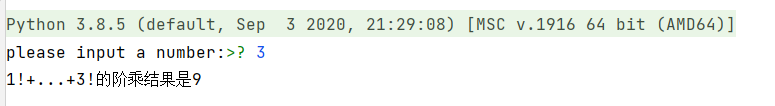• python阶乘怎么写
千次阅读
2020-12-30 04:54:04整数的阶乘(英语：factorial)是所有小于及等于该数的正整数的积，0的阶乘为1。即：n!=1×2×3×...×n。

python阶乘怎么写？

实例#!/usr/bin/python3

# Filename : test.py

# 通过用户输入数字计算阶乘

# 获取用户输入的数字

num = int(input("请输入一个数字: "))

factorial = 1

# 查看数字是负数，0 或 正数

if num < 0:

print("抱歉，负数没有阶乘")

elif num == 0:

print("0 的阶乘为 1")

else:

for i in range(1,num + 1):

factorial = factorial*i

print("%d 的阶乘为 %d" %(num,factorial))

执行以上代码输出结果为：请输入一个数字: 3

3 的阶乘为 6

相关推荐：《Python教程》

更多相关内容
• 在本篇文章里小编给大家整理的是关于Python阶乘求和的代码实例，有需要的朋友们可以跟着学习下。
• 今天小编就为大家分享一篇python 阶乘累加和的实例，具有很好的参考价值，希望对大家有所帮助。一起跟随小编过来看看吧
• ## Python阶乘求和

千次阅读 2021-07-08 11:19:50
依次计算每个阶乘的值，然后相加起来，即可得到结果 代码： # 获得用户输入的整数n，输出 1!+2!+…+n!的值。 num = int(input("please input a number:")) num_sum = 0 for i in range(1,num+1): for j in range(1...

题目：
获得用户输入的整数n，输出 1!+2!+…+n!的值。

思路：
依次计算每个阶乘的值，然后相加起来，即可得到结果

代码：

# 获得用户输入的整数n，输出 1!+2!+…+n!的值。
num = int(input("please input a number:"))

sum = 0
for i in range(1,num+1):
x = 1
for j in range(1,i+1):
x = x * j
sum = sum+ x
print("1!+...+{}!的阶乘结果是{}".format(num,sum))



运行截图：展开全文• Python阶乘求和的方法题目描述：获得用户输入的整数n，输出 1! 2! … n!的值。如果输入数值为0、负数、非数字或非整数，输出提示信息：输入有误，请输入正整数。(推荐学习：Python视频教程)方法一：#factTest1def ...Python阶乘求和的方法

题目描述：

获得用户输入的整数n，输出 1! 2! … n!的值。

如果输入数值为0、负数、非数字或非整数，输出提示信息：输入有误，请输入正整数。

(推荐学习：Python视频教程)

方法一：#factTest1

def main():

a = input()

sum = 0

if a.isdigit():

n = eval(a)

if n > 0:

fact = 1

for i in range(1, n 1):

fact *= i

sum = fact

print(sum)

else:

print("输入有误，请输入正整数")

else:

print("输入有误，请输入正整数")

main()

方法二：递归思想#factTest2

import sys

sys.setrecursionlimit(5000)

def getSum(i):

sum = 0

if i==0:

return 0

else:

for x in range(1,i 1):

sum = fact(x)

return sum

def fact(m):

if m==0:

return 1

else:

return m*fact(m-1)

def main():

n = input()

if n.isdigit():

a = eval(n)

if a>0:

result = getSum(a)

print(result)

else:

print("输入有误，请输入正整数")

else:

print("输入有误，请输入正整数")

main()

问题总结：

当使用递归方法求1024的阶乘时，出现了一个异常：RecursionError: maximum recursion depth exceeded in comparison，超出了递归的最大深度。一些网友提到Python中默认的最大递归深度为1000，但在实际测试中，我的电脑到997的时候就出现异常了，不知道这是由什么决定的。因此，为了能够计算1024的阶乘，需要为最大递归深度赋予一个更大的值。这里可以采用以下方法：import sys

sys.setrecursionlimit(5000) #修改为5000

另外，也可以查看最大递归深度：import sys

sys.getrecursionlimit() # output:1000

展开全文• 我如何去计算python中的一个整数的阶乘？...问题描述 阶乘是我们在很多的数学问题中会遇到的，但是如果我们需要一个很大的数的阶乘，那么自己算起来就会很麻烦，那么我们就能用python来解决这个问题。 让阶乘编程一...广告关闭

腾讯云11.11云上盛惠 ，精选热门产品助力上云，云服务器首年88元起，买的越多返的越多，最高返5000元！

我如何去计算python中的一个整数的阶乘？...

问题描述 阶乘是我们在很多的数学问题中会遇到的，但是如果我们需要一个很大的数的阶乘，那么自己算起来就会很麻烦，那么我们就能用python来解决这个问题。 让阶乘编程一个简单的问题 解决方案我们可以先根据阶乘的定义以及性质写出这样一个简单的程序来解决阶乘问题：? 图2.1简单阶乘以及结果但是这个方式写出来的...

代码：def fei(i,j): #i为行数，j为列数 if i == 1 or j ==1:return 0 elif j == 2 : return 1 else:return fei(i,j-1) + fei(i,j-2) for i in range(1,7):print() for k in range(1,7-i):#控制空格数 print( ,end=) for j in range(1,(2*i)): print(fei(i,j),end=)运行结果：? 以上这篇python递归函数求n的阶乘...

python递归函数谢尔宾斯基三角形python第二十二课：python递归函数常见的递归拍照python第二十二课：python递归函数python递归代码实例递归求阶乘所谓的求阶层，简单的就是12345*6... 一直乘下去非递归版本的函数def fac(n):result = nfor i in range(1,n):result *= ireturn resultn =int(input(请输入您要计算的...

函数内容以冒号起始，并且缩进。 return 结束函数，选择性地返回一个值给调用方。 不带表达式的return相当于返回 none。 语法python 定义函数使用 def ...z #*z返回的是一个元祖 print(a(testing1,testing2)) 输出：(testing1, testing2)再次反过来看下之前的阶乘题目：def jc(n): sum = 1 if n == 1...

函数内容以冒号起始，并且缩进。 return 结束函数，选择性地返回一个值给调用方。 不带表达式的return相当于返回 none。 语法python 定义函数使用 def ...z #*z返回的是一个元祖 print(a(testing1,testing2)) 输出：(testing1, testing2)再次反过来看下之前的阶乘题目：def jc(n): sum = 1 if n == 1...

函数本身可作为参数传递。 python 中使用lambda 来实现匿名函数。 练习1、求n的阶乘def factorial(num): 求阶乘 :param num: 非负整数 :return:num的阶乘 result = 1 for n in range(1, num + 1):result *= n return result n =int(input(n = ))factorial(n)2、实现判断一个数是不是素数的函数。 # 素数，在大于1的...

即如果传入的函数是一个2个数求和的函数，reduce()可以实现累加的结果； 如果传入的函数是2个数求积的函数，reduce()可以实现阶乘的结果。 形式：reduce...python reduce()函数 redeuce()函数是python内置高级函数之一，它与之前介绍过的map()函数类似，同样接收一个函数和一个可迭代对象做参数，返回值是一个值...

usrbinenv pythonimport syssys.setrecursionlimit(5000)#阶乘实现示例：def factorial(n): if n == 1: return 1 else:return n * factorial(n-1)print factorial(3000) 四、递归函数的使用场景：一些场景下循环层次数未知，使用递归会非常简便，例如遍历xml文件节点的代码：#coding=utf-8from xml.dom.minidom ...

如h阶乘，就表示为h!； 阶乘一般很难计算，因为积都很大。 提问：求1+2! +3!+...+20! 的和实现环境：python3编辑器:pycharm分析：1、阶乘的计算就是比较麻烦的一部分，用递归函数实现是比较好的方案，先定义一个递归函数实现求阶乘功能。 defrecursion(n): 定义递归函数实现求阶乘功能ifn==1:return1else:returnn*...一个函数在函数体内部调用自己，这样的函数称为递归函数，递归的次数在python是有限制的，默认递归次数是997次，超过997次会报错：recursionerror.? 一. 递归函数案例案例一：计算数字n的阶乘（举个栗子：9的阶乘 = 9*8*7*6*5*4*3*2*1）# ! usrbinenv python# -*- coding:utf-8 _*-@author:何以解忧@blog(个人博客...

in the funcin the foo()666map()函数map()是 python 内置的高阶函数，它接收一个函数 f和一个list，并通过把函数 f 依次作用在 list 的每个元素上...由于栈的大小不是无限的，所以，递归调用的次数过多，会导致栈溢出#递归实现阶乘n! = (n-1)! × ndef factorial(n): if n == 1: return 1 else:return n *...

函数的默认参数listdir()函数练习：判断输入的是不是一个数字#! usrbinenvpythonimportosdefisnum(s):foriins:ifiin1234567890:passelse:breakelse...即if n == 02）递归参数必须向默认结果收敛，即factorial(n-1)练习：阶乘，n乘以f(n-1)#! usrbinenvpythondeffactorial(n):ifn==0:return1else...

编程语言理论家把“一等对象”定义为满足以下条件的程序实体：在运行时创建能赋值给变量或数据结构中的元素能作为参数传给函数能作为函数的返回结果在python中，整数、字符串和字典都是一等对象，没有什么特别之处。 标题“一等函数”是“把函数视作一等对象”的简称，虽然并不完美，但只是一种称谓。 def factorial...

问题本身很简单，主要是通过这个小问题来演示python的一些用法，例如测试代码运行时间、函数嵌套定义等等。 from time import timefrom math import factorialfrom functools importreducefrom random import randintdef myfactorial1(n):使用传统方法计算阶乘 result = 1 for i in range(1, n+1):result = result*i ...

如果一个函数在内部不调用其它的函数，而是自己本身的话，这个函数就是递归函数。 2.2、递归函数的作用举个例子，我们来计算阶乘 n! = 1*2 * 3 * ... * n...本文标识 : python_8本文编辑 : jack 风编程工具 : anaconda、pycharm阅读时长 :5分钟? 1、引用在python中，值是靠引用来传递来的。 我们可以用id()来判断...

所谓高阶函数，就是可以将函数作为参数输入的一种函数。 在python中，常用的高阶函数有以下几种mapfiltersortedmap的作用和for循环一样，对集合中的每一个元素进行操作，基本用法如# 自定义函数>>> def add(x):return x + 2# 自定义函数作为map的参数，传递进入>>> r = map(add, )>>> r >>> list(r)可以看到，map是一...

如果一个函数在内部调用自身本身，这个函数就是递归函数。 举个例子，我们来计算阶乘 n! = 1 * 2 * 3 * ... * n，用函数 fact(n)表示，可以看出： fact(n) = n! = 1 * 2 * 3 * ... * (n-1) * n = (n-1)! * n = fact(n-1) * n 所以，fact(n)可以表示为 n * fact(n-1)，只有n=1时需要特殊处理。 于是，fact(n)用递归的...python之递归函数好久没有更新内容了，也好久没有给大家打个招呼了，小白想死你们了。 今天跟大家说说python中的递归函数。 python是支持递归函数的。 简单地说，一个递归函数就是直接或间接地调用自身的函数，并且要有退出条件。 枯燥的概念令人生厌，我们直接来个例子看看递归函数是如何工作的。 例如我们对一个...

展开全文• 求1！+2！...是阶乘 定义初始值 a=1 b=1 定义初始结果 c=0 a=int(a) b=int(b) c=int(c) while a<=5:#确定在5以内 b=b*a#阶梯相乘 c=c+b#每个结果相加 a=a+1#计算每个数 print(c)#输出结果
• 整数的阶乘（英语：factorial）是所有小于及等于该数的正整数的积，0的阶乘为1。即：n!=1×2×3×...×n。 <br># 通过用户输入数字计算阶乘 <br> <br># 获取用户输入的数字 <br>num = ...
• 在本篇文章里小编给各位整理的是关于python计算n的阶乘的相关知识点，需要的朋友们参考下。
• python中两种阶乘函数有两种阶乘函数，自己创建的不算。 一是numpy库中的阶乘函数 1 2 improt numpyprint numpy.math.factorial(3) ...
• =0:break print(k) python阶乘函数,实现:输入两个数num1和num2,计算两个数的阶乘并求和_ ： def jc(num): jg = 1 for i in range(1,num+1): jg *= i return jgnum1 = int(input())num2 = int(input())print('{}!...
• def factorial(n): if n==0: return 1 else: recurse=factorial(n-1) result=n*recurse return result def fact(n): s=1 for i in range(1,n+1): s*=...
• 阶乘算法 推导原理 阶乘：n!=123*…n-1n（过于简单，不做过多描述） 代码如下： num=input("请输入一个数：") if num.isdigit(): #天然要求输入的是 >=0 的自然数 num=int(num) result=1 #定义结果初值为1...
• 第三种直接定义一个阶乘函数，随时都可以调用，从而得到不同值。方法一：普通方法：a = 1n = 5for i in range(1,n+1):a = a * iprint(a)20方法二：reduce()函数：from functools import reducen = 5print(...
• 最简单的方法#通过用户输入数字计算阶乘#获取用户输入的数字num = int(input("请输入一个数字: "))factorial = 1#查看数字是负数，0或 正数if num < 0:print("抱歉，负数没有阶乘")elif num == 0or num == 1:...
• print(math.factorial(3)) #3*2=6...